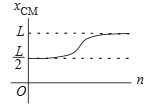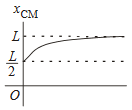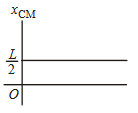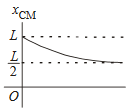#### A thin rod of length L is lying along the x- axis  with its ends at  x = 0  and x = L its linear density ( mass/length ) varies with  x  as k( x/L )n  where  n  can 0 or any positive number. If the position xCM of the centre of mass of the rod is plotted against  n  , which of the following graphs best approximates the dependence of  xCM on n  ?  Option 1)Option 2)Option 3)Option 4)If the rod has the same density as  at x = 0  ie ;  n = 0

therefore uniform, the centre of mass would have been at L/2 .  As the density increases with length, the centre of mass shifts towards the right

Therefore it can only be  (2)

Option 1)It is an incorrect option.

Option 2)It is the correct option.

Option 3)It is an incorrect option.

Option 4)It is an incorrect option.

##### Posted by